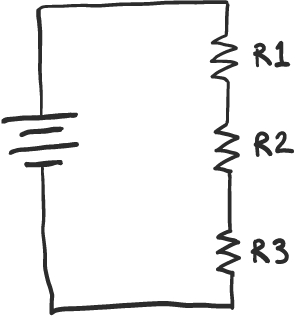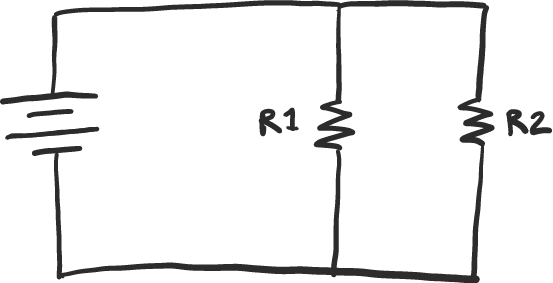Series vs. parallel

## Series connections #

Things that are connected in series are connected end-to-end, like a train.

If you connect a bunch of resistors in series, the resistances add up. All the current has to flow through every resistor, so each resistor restricts the flow more.Above, you see 3 resistors, R1, R2, and R3, connected in series.

If each resistor had a resistance of 1k, and the battery above supplied 6 V to the resistors, how much current would flow?

## Parallel connections #

Things that are connected in parallel are connected such that they share a common input as well as a common output.

If you connect a bunch of resistors in parallel, the total resistance decreases as you add more resistors. If you connect, say, 5 identical resistors in parallel, the total resistance will be 1/5th of a single resistor. The current will split into 5 separate streams, each equal to 1/5th of the total current.Above, you see two resistors, R1 and R2, connected in parallel.

If, as above, each resistor had a resistance of 1k, and the battery above supplied 6 V to the resistors, how much current would flow?